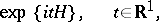# Integral over trajectories

continual integral, functional integral, path integral

An integral for which some function space serves as the domain of integration. Most often, an integral over trajectories is defined as an ordinary Lebesgue integral of a functional defined on a space of (possibly generalized) functions with respect to some (possibly complex) measure in this space. In those cases when the Lebesgue construction of the integral proves to be inapplicable, other methods of functional integration are considered. For example, instead of a measure, a pre-measure (or quasi-measure) can be used, that is, an additive set function defined on the algebra of all cylinder subsets of the function space such that its restriction to any-subalgebra of cylinder sets with fixed support is already a measure. Sometimes integration over trajectories is defined as the limit asof-fold integrals (calculated with respect to the Lebesgue measure in) that arise as a result of suitable approximations of the function space (domain of integration) by an-dimensional space; while the functional to be integrated is approximated by a function ofvariables. These and other definitions of integration over trajectories are applied to their own special classes of functionals; in those cases when these definitions are all applicable, they lead, in general, to different values of the integral. Finally, the integrals over trajectories encountered in physics literature sometimes do not have an exact meaning, but are regarded as formal expressions with which one operates as with ordinary integrals (change of variables, majorization, differentiation with respect to a parameter, limit transitions, etc.); even so, important and heuristically valuable results are often obtained by this means.

Integration over trajectories, which arose originally in the theory of random processes, was subsequently used for representing the groupas well as the semi-group of operatorswhereis a Sturm–Liouville operator in the space(the energy operator for a system of quantum particles). Similar representations were then obtained for a wider class of operators(every representation of this type is usually called a Feynman–Kac formula) and have been an appropriate device for studying the properties of these operators (estimates of the boundary of a spectrum, asymptotics of eigen values, scattering properties, etc., )

Among the applications of integration over trajectories in mathematical physics (based principally on the Feynman–Kac formula), the deepest have proved to be their application to problems of quantum statistical physics  and quantum field theory , . To some extent, the development of general questions of measure theory and integration in infinite-dimensional spaces are related to integration over trajectories .

How to Cite This Entry:
Integral over trajectories. Encyclopedia of Mathematics. URL: http://encyclopediaofmath.org/index.php?title=Integral_over_trajectories&oldid=20470
This article was adapted from an original article by R.A. Minlos (originator), which appeared in Encyclopedia of Mathematics - ISBN 1402006098. See original article